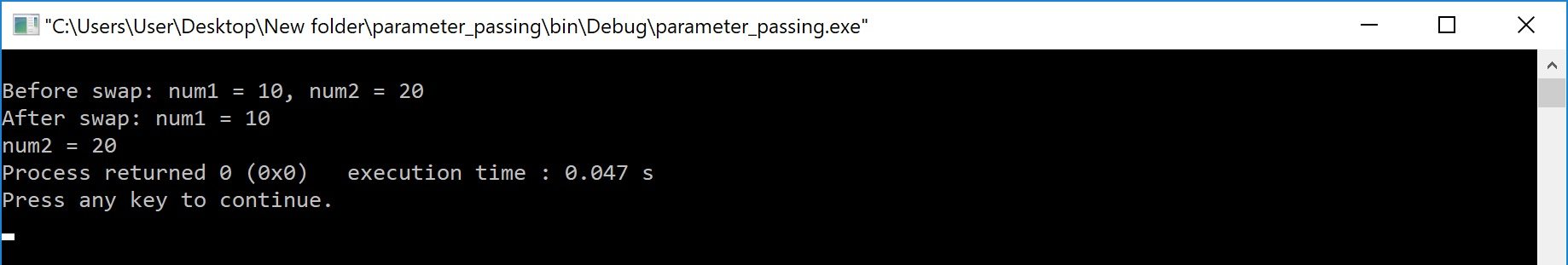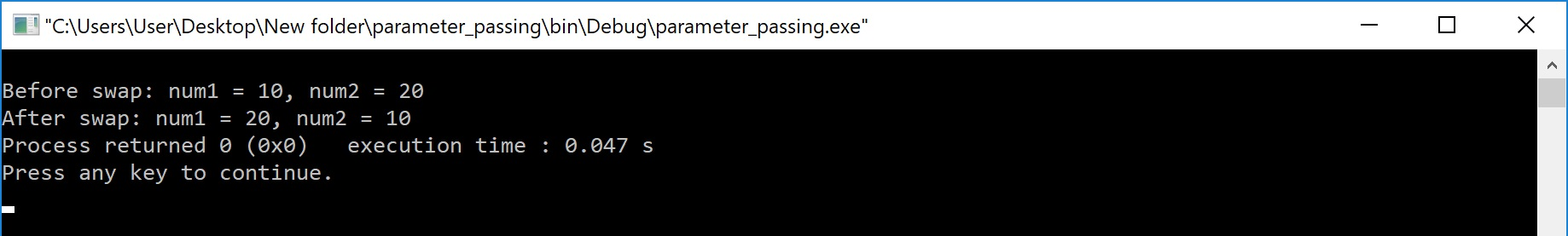The perfect place for easy learning...

×

# Parameter Passing in C

When a function gets executed in the program, the execution control is transferred from calling-function to called function and executes function definition, and finally comes back to the calling function. When the execution control is transferred from calling-function to called-function it may carry one or number of data values. These data values are called as parameters.

Parameters are the data values that are passed from calling function to called function.

In C, there are two types of parameters and they are as follows...

• Actual Parameters
• Formal Parameters

The actual parameters are the parameters that are speficified in calling function. The formal parameters are the parameters that are declared at called function. When a function gets executed, the copy of actual parameter values are copied into formal parameters.

In C Programming Language, there are two methods to pass parameters from calling function to called function and they are as follows...

• Call by Value
• Call by Reference

# Call by Value

In call by value parameter passing method, the copy of actual parameter values are copied to formal parameters and these formal parameters are used in called function. The changes made on the formal parameters does not effect the values of actual parameters. That means, after the execution control comes back to the calling function, the actual parameter values remains same. For example consider the following program...

# Example Program

``````#include<stdio.h>
#include<conio.h>

void main(){
int num1, num2 ;
void swap(int,int) ; // function declaration
clrscr() ;
num1 = 10 ;
num2 = 20 ;

printf("\nBefore swap: num1 = %d, num2 = %d", num1, num2) ;

swap(num1, num2) ; // calling function

printf("\nAfter swap: num1 = %d\nnum2 = %d", num1, num2);
getch() ;
}
void swap(int a, int b)  // called function
{
int temp ;
temp = a ;
a = b ;
b = temp ;
}```
```

Output:In the above example program, the variables num1 and num2 are called actual parameters and the variables a and b are called formal parameters. The value of num1 is copied into a and the value of num2 is copied into b. The changes made on variables a and b does not effect the values of num1 and num2.

# Call by Reference

In Call by Reference parameter passing method, the memory location address of the actual parameters is copied to formal parameters. This address is used to access the memory locations of the actual parameters in called function. In this method of parameter passing, the formal parameters must be pointer variables.

That means in call by reference parameter passing method, the address of the actual parameters is passed to the called function and is recieved by the formal parameters (pointers). Whenever we use these formal parameters in called function, they directly access the memory locations of actual parameters. So the changes made on the formal parameters effects the values of actual parameters. For example consider the following program...

# Example Program

``````#include<stdio.h>
#include<conio.h>

void main(){
int num1, num2 ;
void swap(int *,int *) ; // function declaration
clrscr() ;
num1 = 10 ;
num2 = 20 ;

printf("\nBefore swap: num1 = %d, num2 = %d", num1, num2) ;
swap(&num1, &num2) ; // calling function

printf("\nAfter swap: num1 = %d, num2 = %d", num1, num2);
getch() ;
}
void swap(int *a, int *b)  // called function
{
int temp ;
temp = *a ;
*a = *b ;
*b = temp ;
}```
```

Output:In the above example program, the addresses of variables num1 and num2 are copied to pointer variables a and b. The changes made on the pointer variables a and b in called function effects the values of actual parameters num1 and num2 in calling function.